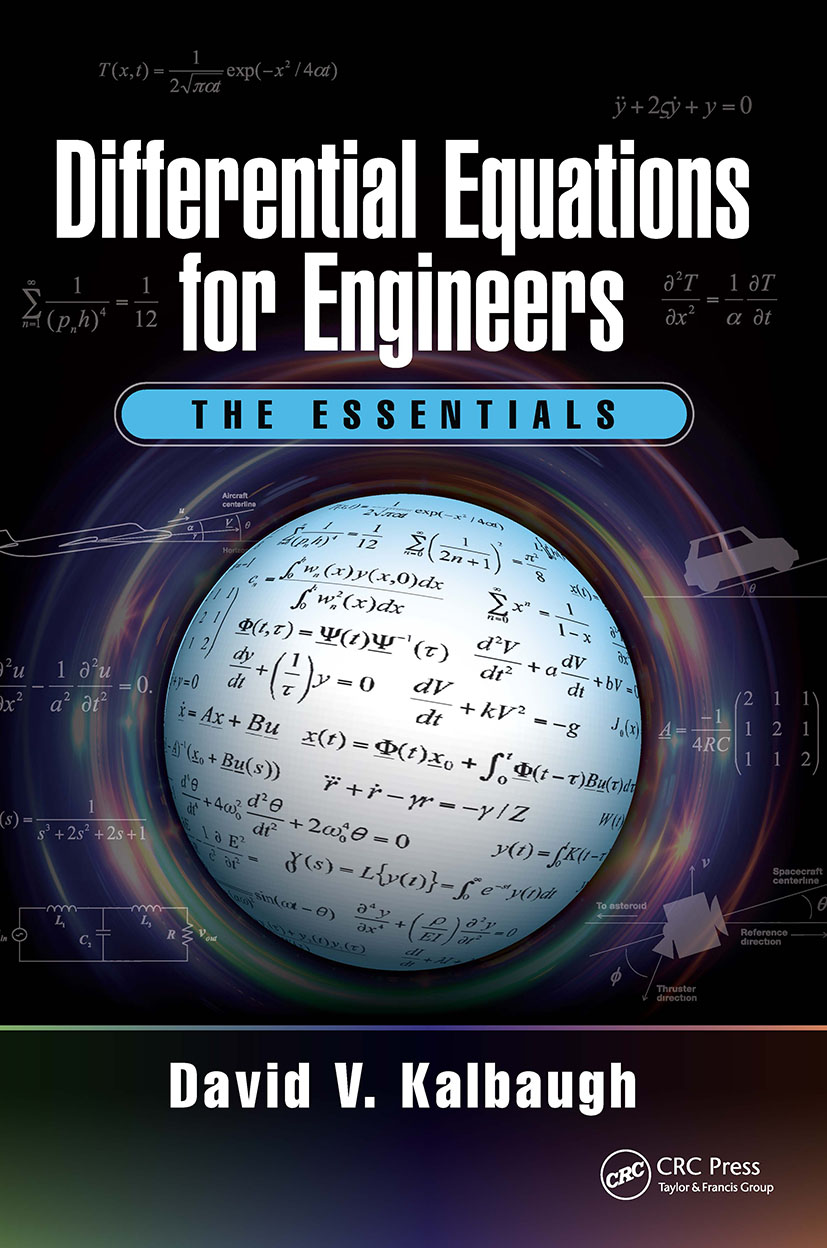# Differential Equations for Engineers

## The Essentials, 1st Edition

CRC Press

433 pages

##### Purchasing Options:\$ = USD
Hardback: 9781498798815
pub: 2017-09-01
SAVE ~\$27.00
\$135.00
\$108.00
x
eBook (VitalSource) : 9781315154961
pub: 2017-09-01
from \$67.50

FREE Standard Shipping!

### Description

This book surveys the broad landscape of differential equations, including elements of partial differential equations (PDEs), and concisely presents the topics of most use to engineers. It introduces each topic with a motivating application drawn from electrical, mechanical, and aerospace engineering. The text has reviews of foundations, step-by-step explanations, and sets of solved problems. It fosters students’ abilities in the art of approximation and self-checking. The book addresses PDEs with and without boundary conditions, which demonstrates strong similarities with ordinary differential equations and clear illustrations of the nature of solutions. Furthermore, each chapter includes word problems and challenge problems. Several extended computing projects run throughout the text.

### Table of Contents

Chapter 1: Introduction

1.1 Foundations

1.2 Classes of Differential Equations

1.3 A Few Other Things

Chapter Summary

Solved Problems

Problems to Solve

Word Problems

Challenge Problems

Chapter 2: First-Order Linear Ordinary Differential Equations

2.1 First Order Linear Homogeneous ODEs

2.2 First Order Linear Nonhomogeneous ODEs

2.3 System Stability

Chapter Summary

Solved Problems

Problems to Solve

Word Problems

Challenge Problems

Chapter 3: First-Order Nonlinear Ordinary Differential Equations

3.1 Nonlinear Separable ODEs

3.2 The Possibility of Transforming Nonlinear Equations into Linear Ones

3.3 Successive Approximations for Almost Linear Systems

Chapter Summary

Solved Problems

Problems to Solve

Word Problems

Challenge Problems

Computing Projects: Phase 1

Chapter 4: Existence, Uniqueness, and Qualitative Analysis

4.1 Motivation

4.2 Successive Approximations (General Form)

4.3 Existence and Uniqueness Theorem

4.4 Qualitative Analysis

4.5 Stability Revisited

4.6 Local Solutions

Chapter Summary

Solved Problems

Problems to Solve

Word Problems

Challenge Problems

Chapter 5: Second-Order Linear Ordinary Differential Equations

5.1 Second Order Linear Time-Invariant Homogeneous ODEs

5.2 Fundamental Solutions

5.3 Second Order Linear Nonhomogeneous ODEs

5.4 Existence and Uniqueness of Solutions to Second-Order Linear ODEs

Chapter Summary

Solved Problems

Problems to Solve

Word Problems

Challenge Problems

Chapter 6: Higher-Order Linear Ordinary Differential Equations

6.1 Example: Satellite Orbit Decay

6.2 Higher Order Homogeneous Linear Equations

6.3 Higher Order Nonhomogeneous Linear Equations

6.4 Existence and Uniqueness

6.5 Stability of Higher Order Systems

Chapter Summary

Solved Problems

Problems to Solve

Word Problems

Challenge Problems

Computing Projects: Phase 2

Chapter 7: Laplace Transforms

7.1 Preliminaries

7.2 Introducing the Laplace Transform

7.3 Laplace Transforms of Some Common Functions

7.4 Laplace Transforms of Derivatives

7.5 Laplace Transforms in Homogeneous ODEs

7.6 Laplace Transforms in Nonhomogeneous ODEs

Chapter Summary

Solved Problems

Problems to Solve

Word Problems

Challenge Problems

Chapter 8: Systems of First-Order Ordinary Differential Equations

8.1 Preliminaries

8.2 Existence and Uniqueness

8.3 Numerical Integration Methods

8.4 Review of Matrix Algebra

8.5 Linear Systems in State Space Format

8.6 A Brief Look at N-Dimensional Nonlinear Systems

Chapter Summary

Solved Problems

Problems to Solve

Word Problems

Challenge Problems

Chapter 9: Partial Differential Equations

9.1 The Heat Equation

9.2 The Wave Equation

9.3 Laplace’s Equation

9.4 The Beam Equation

Chapter Summary

Solved Problems

Problems to Solve

Word Problems

Challenge Problems

### About the Author

David V. Kalbaugh received his B.S. from the Johns Hopkins University in 1967; M.S. from Stanford University in 1968; and doctorate from University of Maryland in 1974. He was exposed to many practical problems across engineering and physics at Johns Hopkins University Applied Physics Laboratory, where he eventually retired as Assistant Director in 2005. Dr. Kalbaugh developed this text while teaching both engineering courses and differential equations as an adjunct Assistant Professor in the Mathematics and Computer Science Department at the University of Maryland Eastern Shore from 2008-2014. UMES achieved ABET accreditation of its engineering program in August 2013.

### Subject Categories

##### BISAC Subject Codes/Headings:
MAT007000
MATHEMATICS / Differential Equations
TEC007000
TECHNOLOGY & ENGINEERING / Electrical
TEC009070
TECHNOLOGY & ENGINEERING / Mechanical